# 40 + 40 x 0 + 1= ?

Long answer part 1: is this to be solved by parsing or by algebra? If it's to be solved by parsing, we need a set of parsing rules, in other words a convention. Grade school teaches things like BEDMAS/PEMDAS, but that's a fairly complex rule operating on groups. Instead let's go with one particular way of computer program parsing that is easy for a beginner programmer to write. The general algorithm goes like this:

Read the first number until the operator is found. Create a tree leaf containing the operator, with a left branch containing the first number read, and a right branch being empty. Read the next symbol: if it's a parenthesis, start over but with the right branch becoming a new "leaf" to hold the next operator. If it's another number, put it into the right branch. Now simplify by applying the leaf operator to both its branches, and storing the result inside the leaf and clipping the branches. Read the next symbol, if it's an operator create a leaf with a left branch containing the resulting value previously computed and a right branch containing nothing... repeat.

Step 0: read in our expression, "40+40x0+1", and put each distinct element in its own box.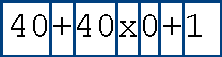Step 1: start in the first box, read the value. "40". It's a number, so create a new "leaf" and put "40" inside.Step 2: read the value in the second box. "+". It's an operator, so create a new, bigger "leaf" and put "+" inside, then create a child branch to the smaller leaf with 40.Step 3: read the value in the third box. "40" again. Put it in a small leaf and add a second branch from the "+" to it.Step 4: pause. The "+" has two child branches, so we must simplify. Perform "+" on the two leaves, so "40+40", which is "80", put "80" in the leaf where "+" was, and get cut off the old branches.Step 5: continue and read the value in the fourth box. "x". It's an operator for multiplication, so create a big leaf and put "x" in it, and create a branch to "80".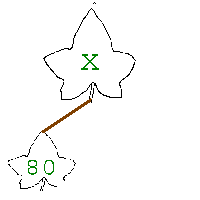Step 6: read the value in the fifth box. "0". Put it in a leaf that is connected by a branch to the "x".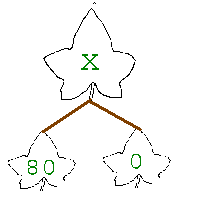Step 7: pause again, we have to simplify. Compute "80x0", which is 0, and put it in its own leaf.Step 8: read the value in the sixth box. "+". Same story: big leaf, put "+" in it, create a branch that connects it with "0".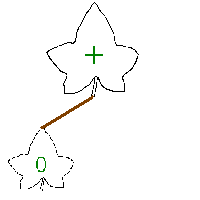Step 9: read the last value. "1". It becomes a leaf with a branch from the "+".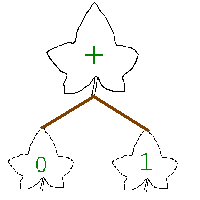Step 10: simplify for the final answer. "0+1" is 1.

Since according to rules like PEMDAS the answer should be 41, not 1, we say the above expression was "malformed". (Alternatively you might say our method of parsing is inadequate for not guessing what we mean.) You can get the right answer in this parsing system by adding parentheses in the right places, or you can use a more complicated parser that conforms to PEMDAS.

Long answer part 2: Why do we want PEMDAS's answer anyway? The answer comes from algebra. $40 + 40*0 + 1 = x$ is the algebraic question, which we must apply transformations to using algebraic rules. The particular rules are chosen in the same way that the particular parsing method is chosen--because we want the answer to be a particular something. In this case, we can use real-valued definitions and axioms of a vector space. The definitions describe addition and multiplication as operations on two numbers. The associativity axiom lets us rewrite as $40 + 1 + 40*0 = x$. Applying the definition of times, we rewrite as $40 + 1 + 0 = x$. Applying the definition of plus, we reduce to $41 + 0 = x$. Applying the definition of plus again, we reduce to $41 = x$.

Note that the definition of scalar multiplication implicitly takes precedence over addition. From $40 + 1 + 40*0 = x$, we might have said $41 + 40*0 = 81*0 = 0 = x$. But from the definitions, "40+1" constitute two distinct elements "40" and "1", while "40*0" does not--it constitutes one distinct element "x" in X, which in this case is "0". So the road to $x=0$ would be erroneous within our vector space axioms. The associative transformation above was also unnecessary since that multiplicative substitution takes precedence regardless (just like PEMDAS says), it's there just for fun.

#### Posted on 2011-10-16 by Jach

Tags: fodder, math

Back to the top

Anonymous November 10, 2011 02:37:24 PM The answer is actually 1. Anything multiplied by zero can't be multipled at all. Which is why all times tables start off with 1x2=2 :)
Jach November 10, 2011 07:37:12 PM Not sure if serious or trolling... =P
Sarah November 11, 2011 06:47:10 AM Joe bloggs say 1, Mathematicians say 41!!! :(
Shaun April 03, 2012 06:59:12 AM 40 + (40 x 0) + 1 = 41 - I think we can all agree on that. The parentheses guide us to the correct answer.

However, many people seem to be insisting that 40 + 40 x 0 + 1 (should also) = 41 ... because we should "assume" the parentheses, when no such indication exists.

It is clearly a malformed calculation and *should* include the parentheses for clarity. Having done so would have saved millions of characters from being typed into blogs, forums and social networks!! lol
John April 03, 2012 09:58:02 AM Shaun - I agree entirely. Therefore without the brackets the answer is 1, you should never 'assume' anything. You should go off what is put in front of you. FACTS! How can you assume that there should be brackets there? Remember to ASSUME makes an ASS out of U and ME!
Jach April 03, 2012 04:59:26 PM Shaun and John: math relies on assumptions, as does deductive logic in general. You say it's a malformed expression, every programming language disagrees with you.

For instance, if you type into Python:

>>> 40+40*0+1
41

And as my long answer explains, if you pick an axiom system you can still arrive at the correct answer without needing parentheses to clean up the expression. We only assume the axioms, we don't assume the parentheses--reread my post. (It's depressing that this is the most popular post I have and that it's still misunderstood.) I proved that the expression is not malformed in the vector space axioms (admittedly it could be a tighter proof), I could pick a different set of axioms as well. It's only a malformed expression when your parser gives you the wrong answer--the naive parser algorithm I outlined above does, but every actual programming language I've ever seen (and I've seen a lot) uses a more sophisticated parser to get the right answer.
Anonymous April 05, 2012 06:31:00 PM Multiply, divide, add, subtract...that is the order if there is no parenthesis or brackets. Elementary school education.
Jach April 05, 2012 06:41:52 PM Oh you. I wonder if there are any reading-comprehension-quiz programs out there to force people to pass before commenting...
GFS June 11, 2012 03:33:29 PM LOL Jach! Jach is on the money! Correct me if I'm wrong ... if one is using a simple calculator, they would see the result of 1. Simple calculators were not designed to do complex calculations but instead use 'Polish' notation. If you were to use a more sophisticated calculator, you would type in the complete problem and see the result of 41 ... which is the correct answer. Even MS EXCEL calculates correctly because it was designed to do complex calculations. Put the following into a cell ... '=40+40*0+1' and hit enter ... the result is 41!
Jonathon September 23, 2012 12:40:14 PM Anonymous is actually right... If you get really deep into the theoretical parts of math multiplication by 0 yields no definite number (never EXACTLY 0, but something infinitesimally close to 0). This is because no number can be 100% precise - it can be off by a one-quadrillionth. That's why division by 0 is a mathematical no-no. In the case of division by 0 it would result in an incomprehensible large number that is just easier to label as "undefined." Anyway, Anonymous is correct. Although if one were to view the problem more simply, the answer would be 41.
Jach September 23, 2012 04:48:01 PM Jonathon, we can assume that the numbers in question are elements of the Reals. All arithmetic operations thus do in fact yield definite numbers, even ones including 0 (a Real number).

Division by 0 is a separate issue. We call it "undefined" because, without further information, division by 0 implies both positive and negative infinity, which aren't elements of the Reals but behaviors of the Reals. Sometimes we have extra information, such as which direction the division is coming from. That is, if we are taking the limit as x goes to 0 of 1/x, it's undefined. But if we know also that x goes to 0 from the positive direction, we know the answer is positive infinity.

Infinity isn't an incomprehensibly large number, it's a behavior of numbers. An incomprehensibly large number is, say, Graham's Number.

Now if we do move into the Hyperreals, things change a little. 0 is indeed the only Real that becomes infinitesimal, all others remain finite. 1/0 remains undefined. We're allowed to write down numbers of the form "2.000...1" with infinitely many 0s, which outside of the Hyperreals makes no sense because you can't have infinitely many of something followed by finitely many of something else. Hyperreals lets us formalize infinities as elements of our set. I haven't studied much practical purpose for the Hyperreals, only the theory, though I do submit to the common argument that the concepts of differential and integral calculus are much easier to grasp with this system than with the usual system of limits, and that the epsilon-delta method needs to die. (I have fruitfully studied the Surreals which are quite useful in the practical analysis of combinatorial games.)

Unfortunately for your argument, we're still using standard arithmetic. Even if our numbers here are elements of the Hyperreals, all theorems that apply to the Reals equally apply to the Hyperreals. As my argument is based on vector space axioms (which are theorems of other axioms), the answer of 41 stands. You can get as complicated and deep into mathematics as you like, the answer will continue to be 41.
Anonymous September 24, 2012 12:00:21 PM All I want to say is that jach, you really know your sh*r. So much that most people can't follow you, have no idea what axiom or parsers are and do not comprehend what you are saying at all. Its funny to me how most of these people clearly have a very basic understanding of math and are probably going 100% off of something someone else said or something they've read. With no actual knowledge or comprehension of extremely complex math solutions.all you can really do at this point is suggest that these people take some actual classes and educate themselves, as they should before spreading suggestive ignorance or limiting most individuals by simplifying complex solutions, otherwise its going to be you who is going to have to continue to break this shit down more and more until its finally basic enough that most can understand and that my friend, is going to take you a very long time as I'm sure you didn't learn all this over night or by someone telling you.
Jach September 24, 2012 01:53:45 PM Thanks for the comment Anon. I updated the post with an easier picture-based way to understand the example parsing algorithm (that gets the wrong answer!) so hopefully that helps someone.
PURPLEBUGG September 24, 2012 08:40:07 PM What I learned in elem. Was to use pemdas; parenthesis, exponets, multiplication, devisionm addition, subtracction. Then in Algebra 2b honors I learned. Parenthesis (ANY kind of brackets), Exponents, Mulitplication and devision (which ever one comes first from left to right), addition and subtraction (which ever one comes first from left to right)
Alyssa April 08, 2014 12:27:13 AM look, i see people talking about parenthasees, or brackets. but in the stated equation there are none. so you assume there are none exactly as it looks and do the equation; it reads 40+40x0+1= which means you first do 40X0 according to order of opperations multiplication/division always comes before addition/subtraction after getting that 0 all that remains is adding the other 40 and the 1... yeilding. le`gasp... 41! its not rocket science and even if it was, thats not hard either. i assume the whole point of Jach's posting this equation was to point out the intricacies of programming and complex mathematics which yes, unless a program is specfically coded to look for and perform the order of opperations, or simplify the code to "emulate" the order of opperations it will 100% of the time do the equation linearly as it is writen instead of properly using the order of opperations
Yankee July 16, 2014 11:08:35 PM http://m.youtube.com/watch?v=RF2W755inko
Anonymous August 12, 2018 06:12:24 AM 6÷2(1+2)=

Comment using the form below

(Only if you want to be notified of further responses, never displayed.)

LaTeX allowed in comments, use $\\...\\$\$ to wrap inline and $$...$$ to wrap blocks.